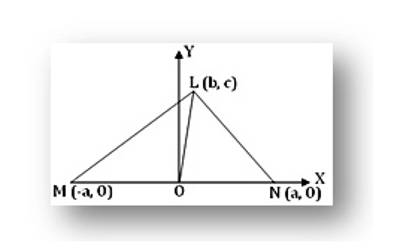Apollonius’ theorem is an elementary geometry theorem relating the length of a median of a triangle to the lengths of its sides. It states that “the sum of the squares of any two sides of any triangle equals twice the square on half the third side, together with twice the square on the median bisecting the third side”. Apollonius’ theorem is proved by using co-ordinate geometry. Proof of this geometrical property is discussed with the help of step-by-step explanation along with a clear diagram.Statement of the Theorem: If O be the mid-point of the side MN of the triangle LMN, then LM² + LN² = 2(LO² + MO²).

Proof: Let us choose origin of rectangular Cartesian co-ordinates at O and x-axis along the side MN and OY as the y – axis. If MN = 2a then the co-ordinates of M and N are (- a, 0) and (a, 0) respectively. Referred to the chosen axes if the co-ordinates of L be (b, c) then

LO² = (b – 0)² + (C – 0)², [Since, co- ordinates of O are (0, 0)]

= b² + c²;

MO² = (- a – 0)² + (0 – 0)² = a²

LM² = (b + a) ² + (c – 0)² = (a + b)² + c²

And LN² = (b – a) ² + (c – 0) ² = (a – b)² + c²

Therefore, LM² + LN² = (a + b) ² + c² + (b – a)² + c²

= 2(a² + b²) + 2c²

= 2a² + 2(b² + c²)

= 2MO² + 2LO²

= 2(MO² + LO²).

= 2(LO² + MO²). Proved.

Information Source: